# How to Teach the Doubles Facts So Your Students Actually Understand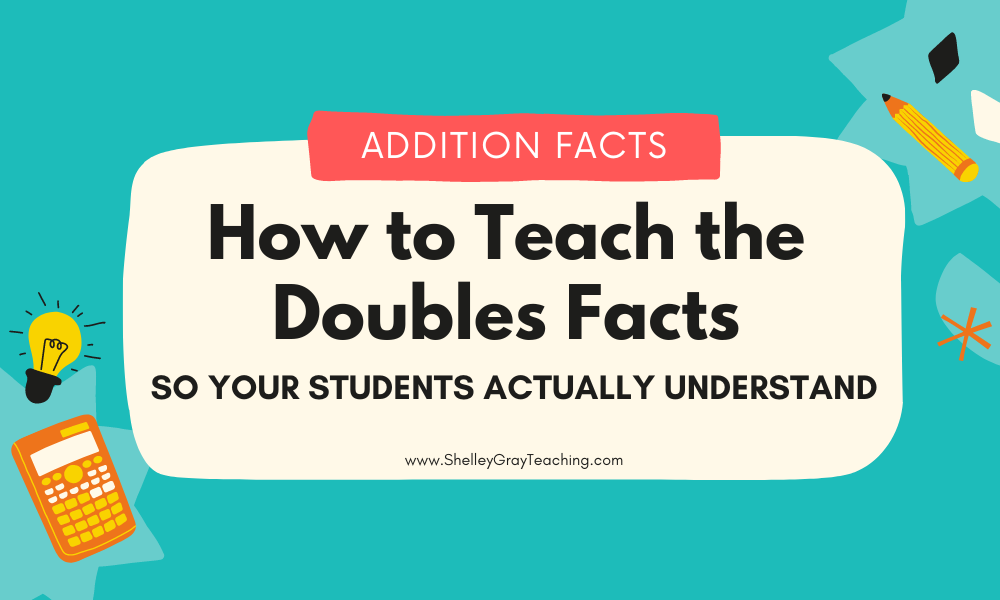What are the doubles facts in math? How do you teach them? Why are the doubles addition facts important? By the time you finish reading this article, you’ll have an excellent understanding of all things “doubles” and also leave with some free resources and fun games to get you started teaching this important math concept in your classroom!

## WHAT ARE THE DOUBLES FACTS?

Doubles refers to adding the same number twice, for example 5+5. Generally when we refer to the doubles addition facts in elementary math, we are referring to facts from 1+1 to about 12+12.

The doubles are not so much a math strategy as a set of facts that students should know. When kids know the doubles, they are able to use them as a building block for other mental math strategies such as near doubles. But that’s another article.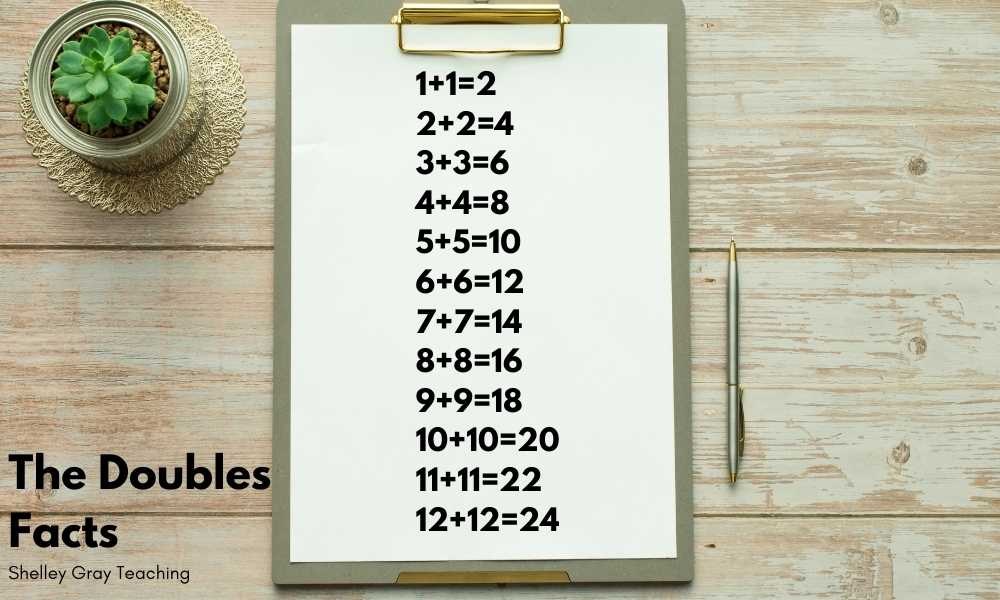## THE DOUBLES FACTS IN REAL LIFE

Doubles are generally an easy set of facts for students to remember because they are all around us in real life. Think of the wheels on a car (2+2), the legs on a spider (4+4), fingers and toes (5+5), or the eggs in a carton (6+6).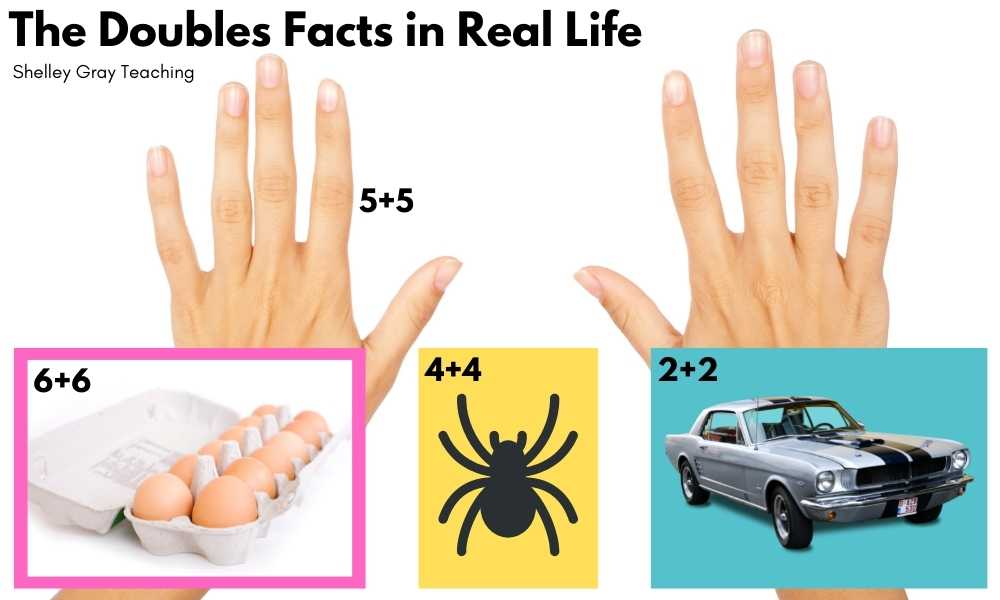## REMEMBERING THE DOUBLES FACTS

Your students will have an easier time with more advanced mental math strategies and even with multiplication by 2 if they just know the doubles math facts. But that doesn’t mean you have to resort to memorization only.

It is important for students to work with math facts in a hands-on way as they build their understanding.

One of the best ways to work with the doubles facts is with ten frames. Have your students build the fact on the ten frames with different colors.

You can also have students build doubles using a rekenrek, or even simple colored cubes.## To understand why the doubles math facts are important, it helps to have a vision for where you are headed. Here are some other concepts that rely on the doubles.

### NEAR DOUBLES

Near doubles are facts like 5+6, 10+11, or 3+4. For these facts, we can use what we know about doubles to solve a new fact. For example, for 3+4, we can think, “I know that 3+3=6, so 3+4 is just one more!”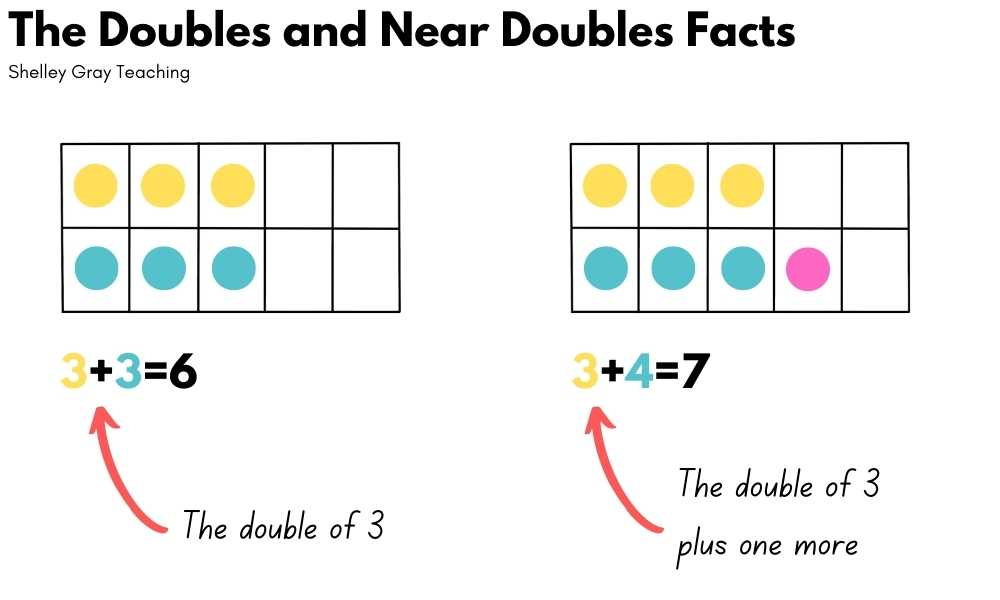### MULTIPLICATION BY 2

In third grade when students learn to multiply, the 2 times table is generally the first set of multiplication facts they learn. We teach these facts by making a connection to the addition doubles. For example, to solve 2×3, we can think, “2 groups of 3” or 3+3.## DOUBLES FACT PRACTICE

There are lots of simple ways to practice the addition doubles, but some of my favorite involve games.

The first one is called Dice Doubles. This takes 1 minute and is something I used to do with my classes all the time to increase automaticity. My favorite part of this game is that each student is only competing against themselves, trying to increase their own score each time.

The second game is another dice game called Doubles Race to 100. It’s a fun partner game that is sure to raise the energy level in your classroom!

## FREE DOUBLES FACT BOOKLETS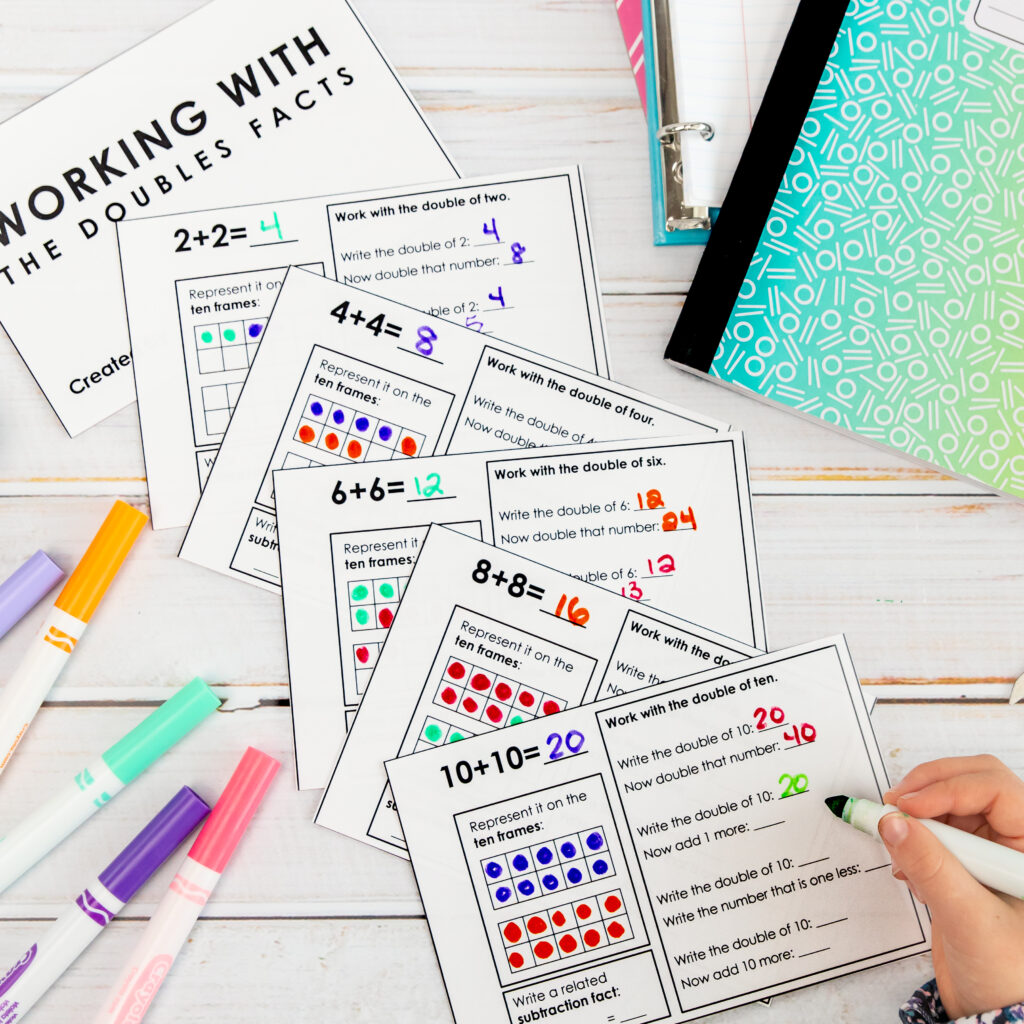These booklets will be fun for your students to complete. Not only do they focus on the addition doubles, but also on how we can make different connections using 1 more, 1 less, 10 more, and repeated doubling!

Get this free booklet here.

This site uses Akismet to reduce spam. Learn how your comment data is processed.